Remember Me

Create an account

Fields marked with an asterisk (*) are required.
Name *
Email *
Verify email *

AFT Product Tips

Font size: +
4 minutes reading time (778 words)

Calculating Pressure Drop Across Sharp-Edged Perforated Plates

Sometimes design engineers will install a screen to protect equipment like centrifugal pumps. Screens are available in a variety of configurations and each has its own head loss characteristics. As such, this article will strictly discuss thin-walled perforated plates with uniform diameter holes. AFT Fathom provides a screen junction that allows users to choose from four different screen types; Perforated Plate (Sharp-Edged), Perforated Plate (Round-Edged), Circular Wire, and Silk Thread Screen.

Every screen has an open flow area that allows the working fluid to bypass the screen while restricting any foreign objects that are not able to pass through this area. The open flow area is typically comprised of several apertures that are evenly distributed across the fitting. Industry sometimes refers to the flow area as porosity. To determine the flow area percentage of the screen, it is broken up into discrete segments, usually on a center point basis between openings. These segments are then dimensioned, along with the open areas as well. The open area ratio (porosity), f, can be calculated by dividing this open area of the segment by the total area of the segment.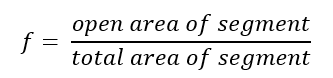There are several common geometries for perforated plates. These may have elongated geometries or simple round holes. The principle outlined above works with all types to determine an open percentage ratio. While the calculations may be easy enough to compute by hand, three circular configurations have been outlined below to save time. There are several tools also available online to help with calculating the open flow area ratio for various geometries. However, users should verify the calculations presented on these websites. When researching information for this blog article, it was determined that several of these websites used some incorrect formulas.

Perhaps the three most common layouts are as follows; the square arrangement, a staggered profile, and a staggered profile where openings are offset by 60 degrees. Note, the staggered profile will work for the 60-degree offset as well, it may be faster however to use the simplified equation. These three layouts are shown below.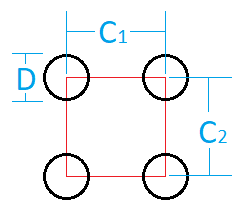Figure 1 Square arrangement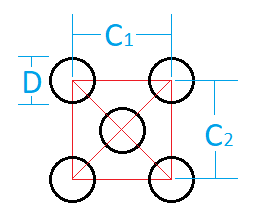Figure 2 Generic staggered arrangement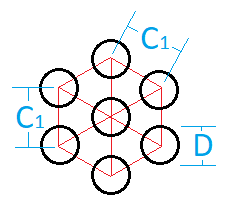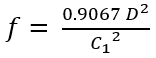Figure 3 60-degree offset staggered arrangement

Once the open area ratio is determined, this can be used in the following equation. This is listed in Idelchik’s Handbook for hydraulic Resistances, 1994 on page 516. The equation results in a k-factor which is then converted directly into a pressure drop as with most k-factors shown below.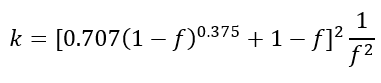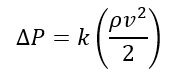AFT Fathom uses the above equation to determine the pressure drop across the screen junction when the Perforated Plate (Sharp-Edged) option is selected. Coupled with the area of the pipe, upstream by default, the input flow area will be used to determine the open flow area ratio across the screen. Figure 4 below shows an empty Screen Properties window. In addition to the elevation of the screen, the flow area is required to accurately determine the loss across the screen.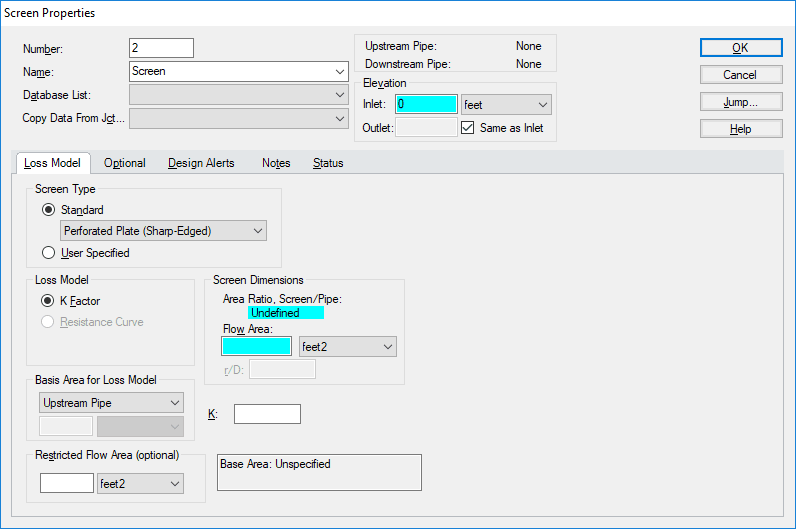Figure 4 Screen Properties window

Users will typically have access to the configuration information such as the staggering, spacing, and diameter of the holes on their perforated plates. As such, the flow area must be calculated by hand to be input into AFT Fathom. The equation below handles this directly.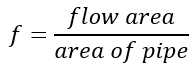Consider an example for a perforated plate with sharp edges like the one shown below. The holes resemble a square layout and are spaced out every 0.5125 inches and have a bore diameter of 3/8 inches. The screen is to fitted to a nominal five-inch steel pipe (hydraulic diameter: 5.047 inches). What would the k-factor be for this screen?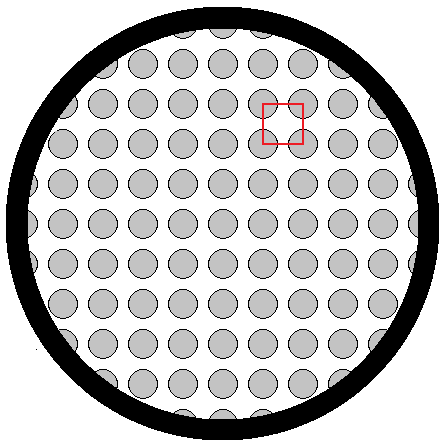Figure 5 Example screen

To begin, one would determine the open area ratio of this screen using the following equation. Substituting the measured values into the equation as shown, the value is determined to be 0.42045. The resulting flow area is then, 8.411373 square inches. This is then input into the Screen Properties window. Users should then verify the calculation by comparing the resulting open area ratio with that reported by AFT Fathom. Figure 6 shows the Screen Properties window with the above information entered. The k-factor in this case is 7.55642.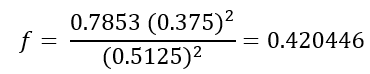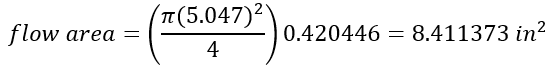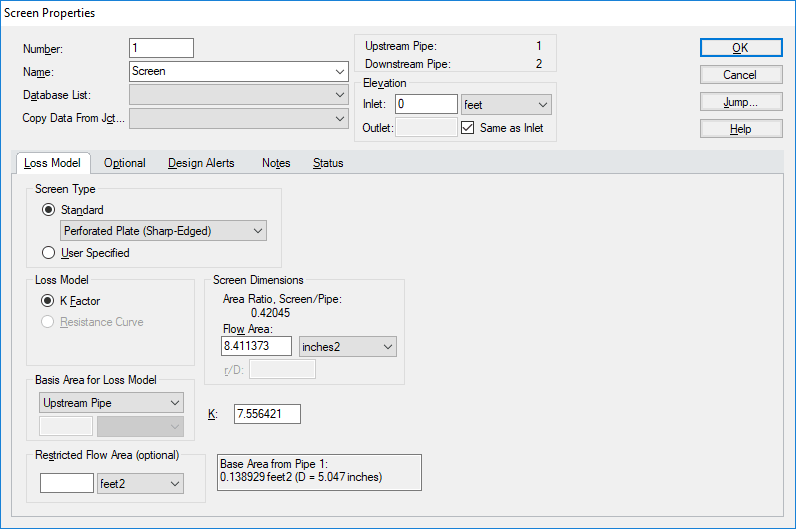Figure 6 Screen Properties window filled out

It is easy to accurately predict the pressure drop across a perforated plate with sharp edged orifices as shown above. Be mindful however, that the characteristics discussed above pertain to thin-walled plates. As plates become thicker, the frictional dissipation across the openings begins to resemble a tube bundle and as such, the equations used to characterize this loss should change as well. There are other equations for both round edges and different methods to determine the open area ratio for irregular shapes and staggering patterns.

Related Posts

Aaron Ren on Wednesday, 27 December 2017 01:44